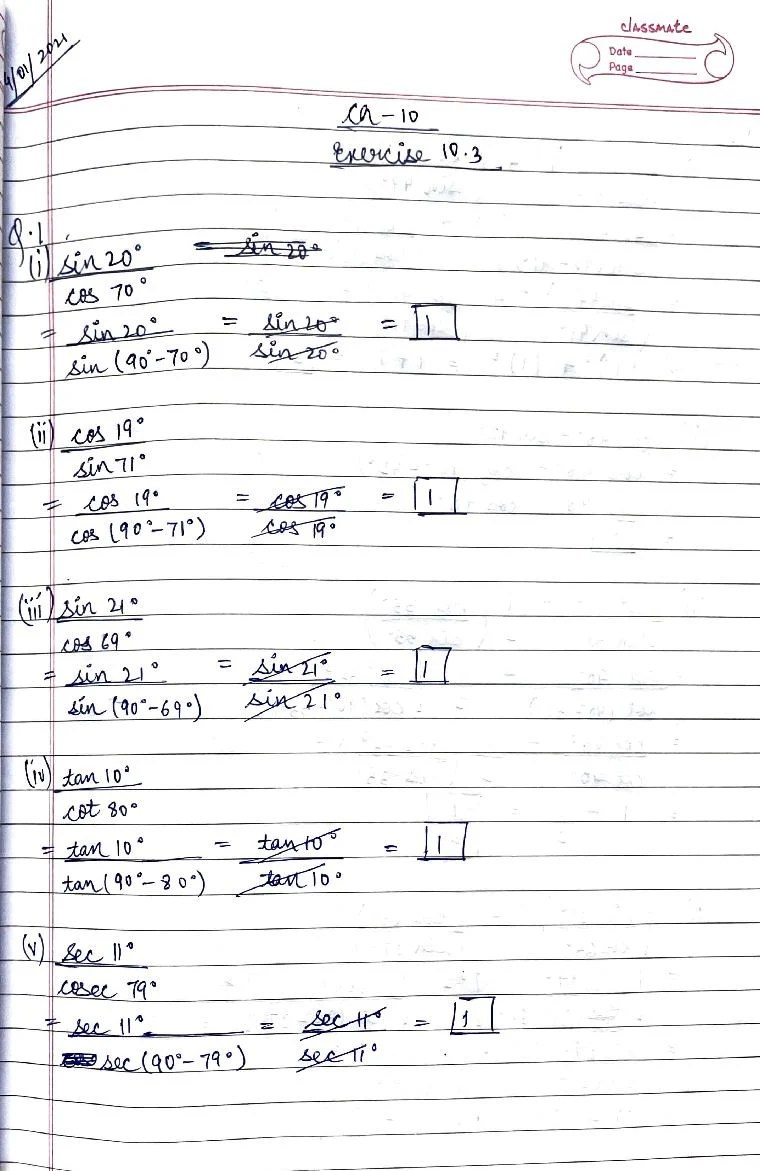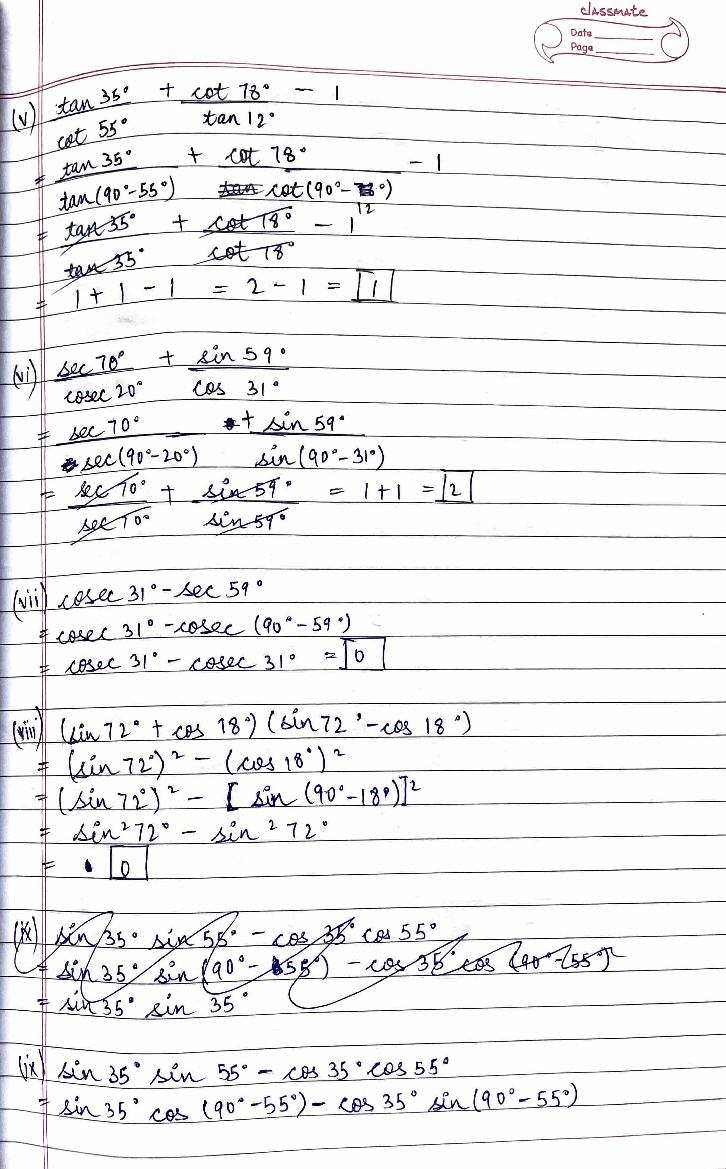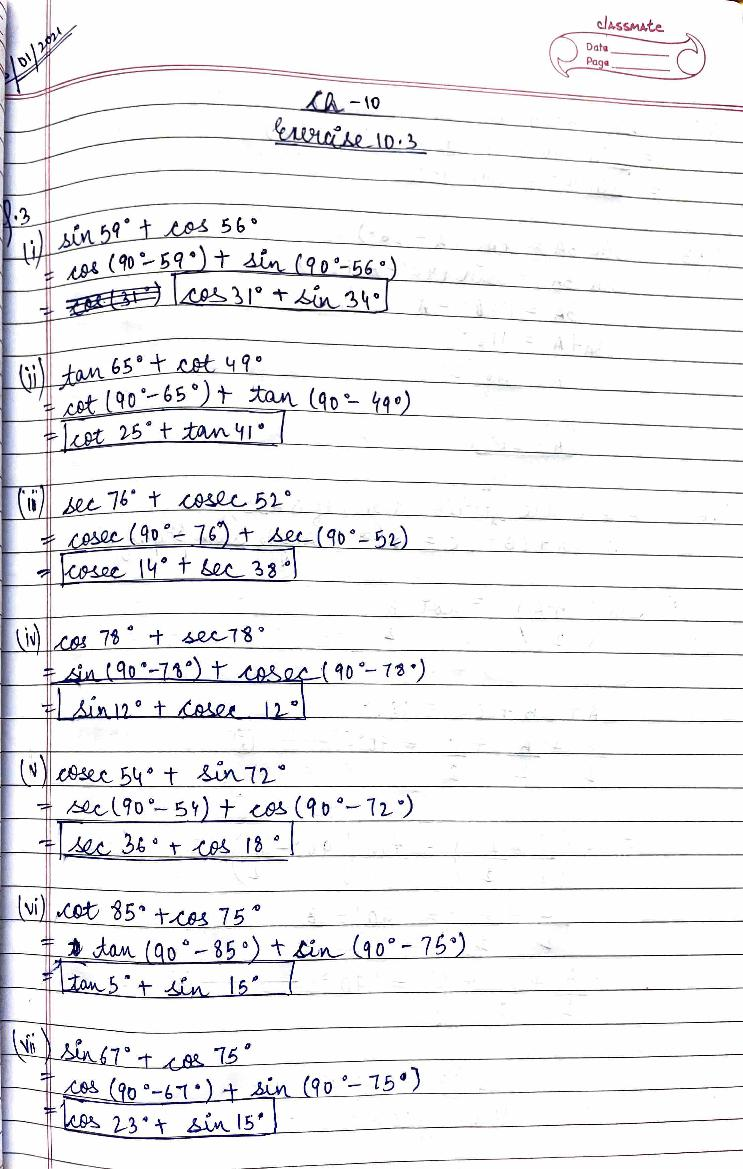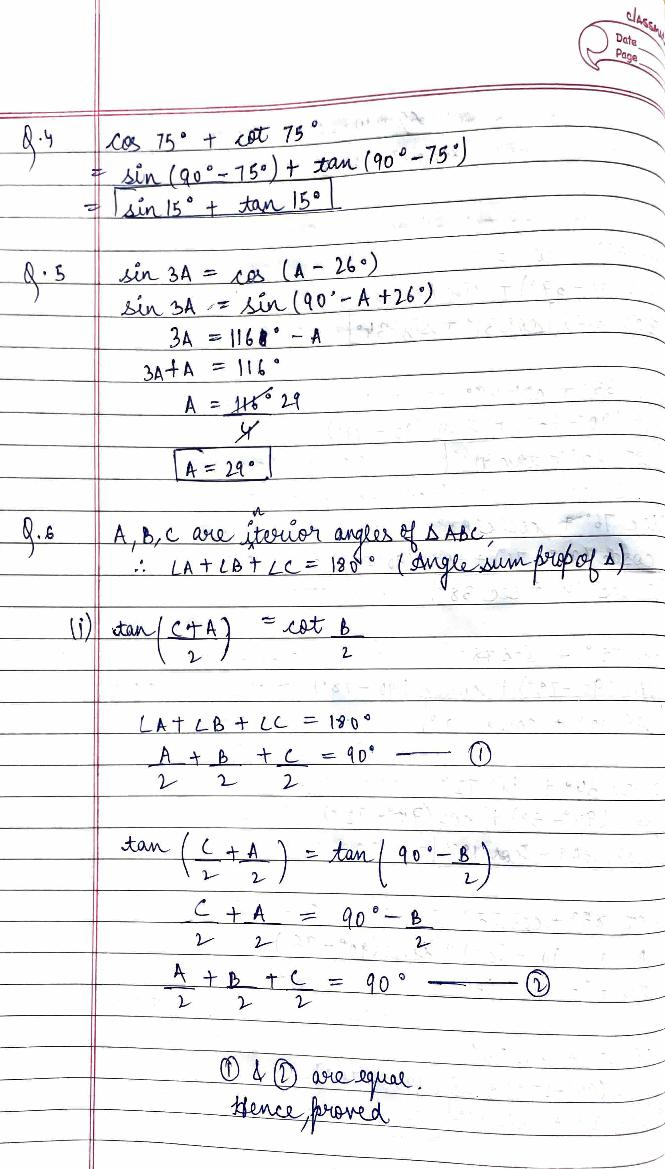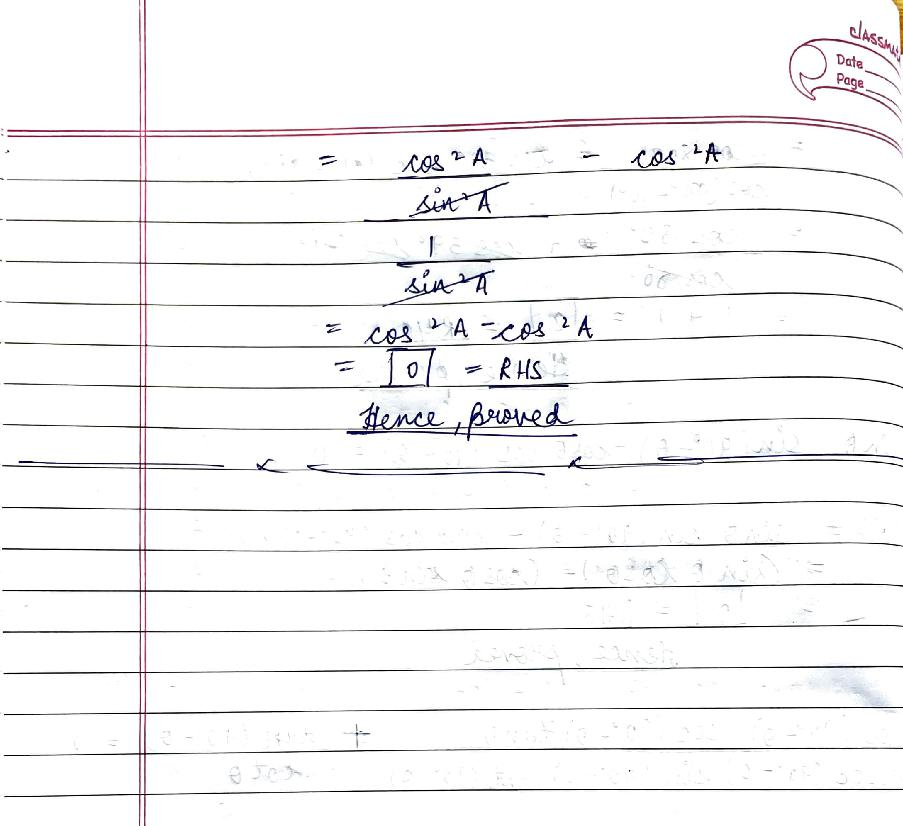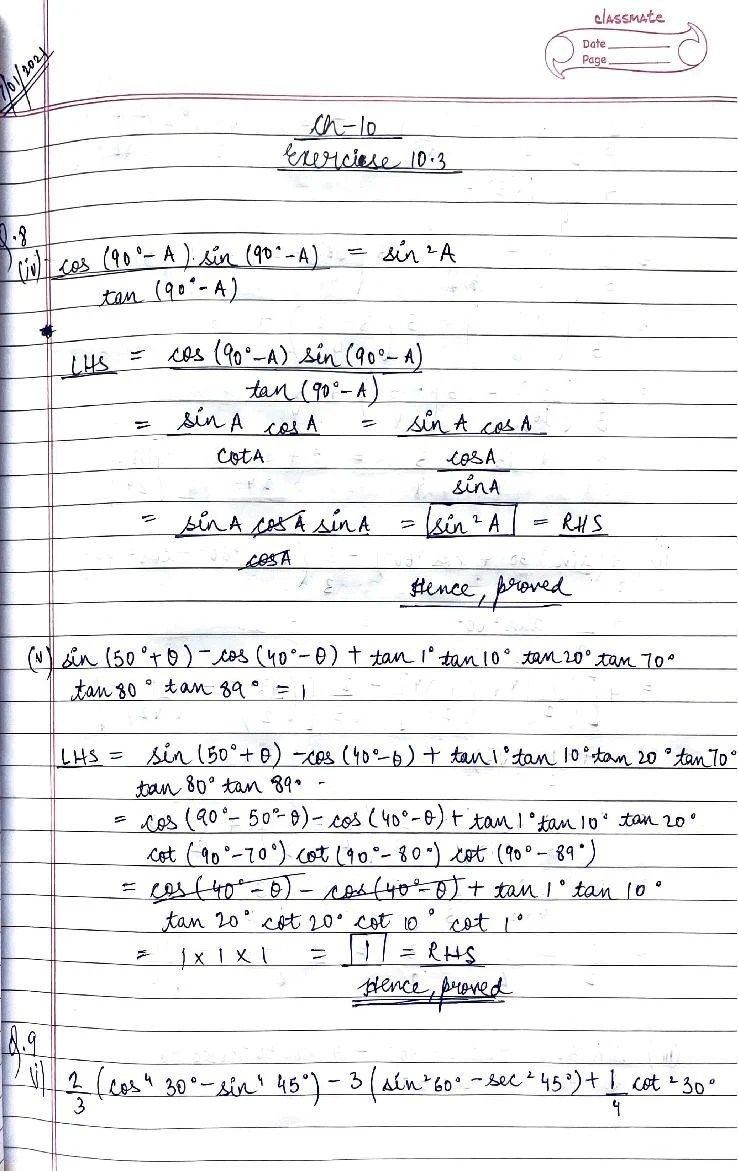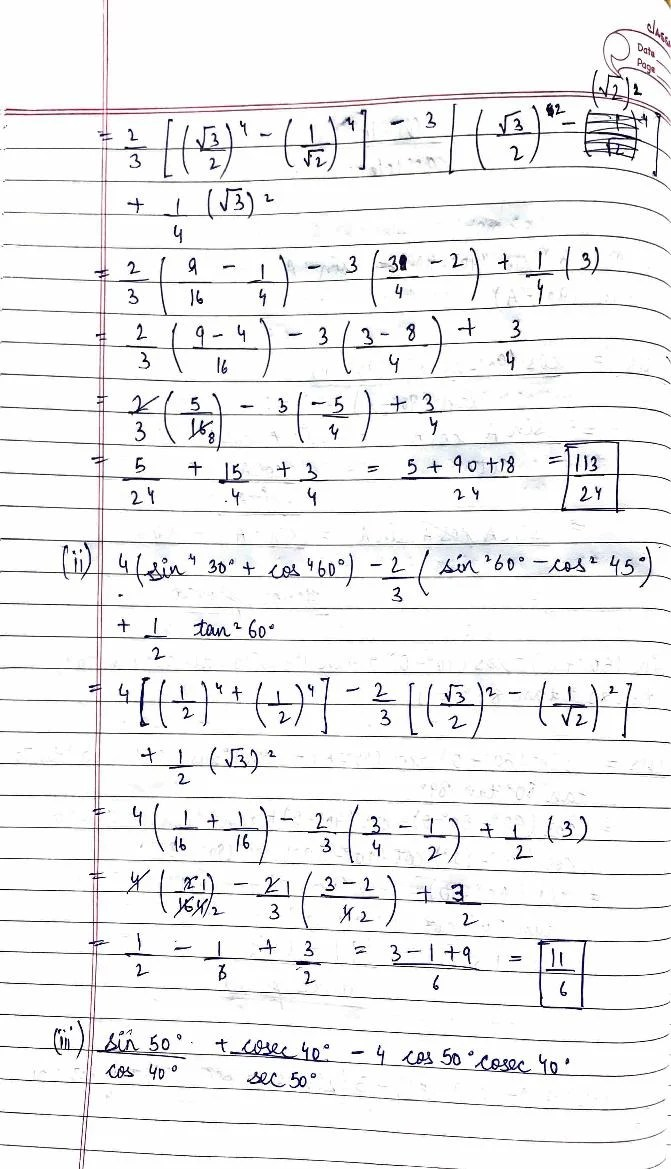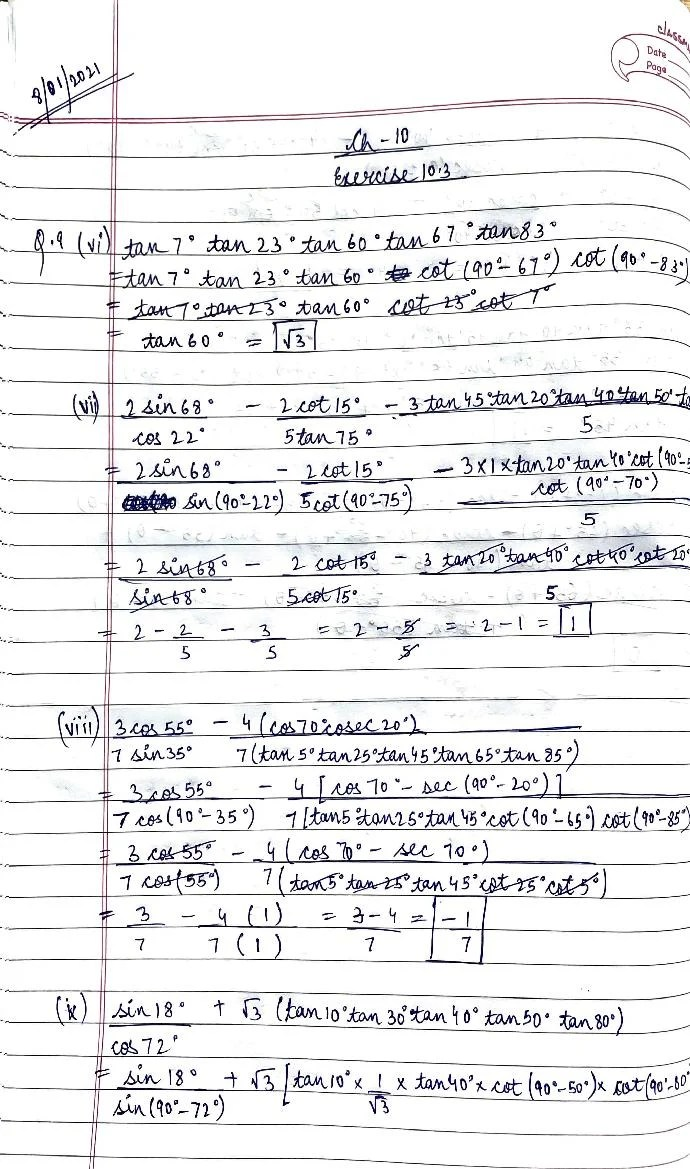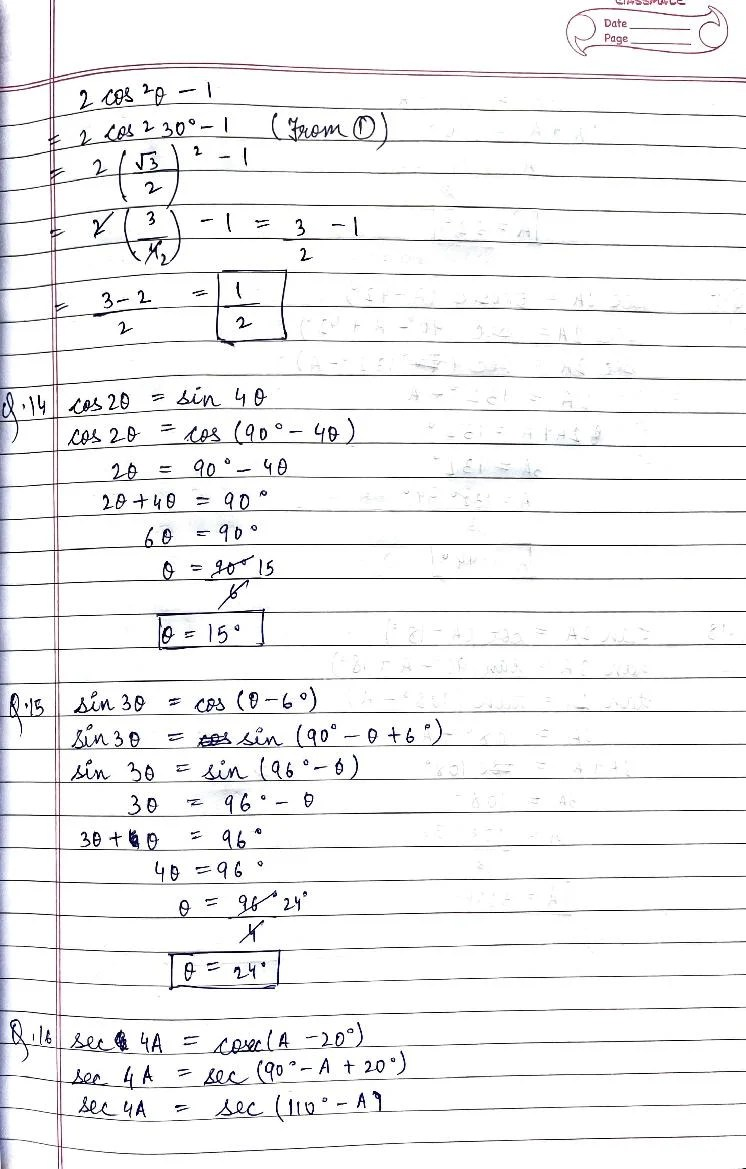# RD Sharma Solutions Class 10 Chapter 10 Trigonometric Ratios Exercise 10.3

Here you can get free RD Sharma Solutions for Class 10 Maths Chapter 10 Trigonometric Ratios Exercise 10.3. All RD Sharma Book Solutions are given here exercise wise for the chapter Trigonometric Ratios. RD Sharma Solutions are helpful in the preparation of several school level, graduate and undergraduate level competitive exams. Practicing questions from RD Sharma Mathematics Solutions for Class 10 Chapter 10 Trigonometric Ratios is proven to enhance your math skills.

 Class: Class 10th Chapter: Chapter 10 Exercise: Exercise 10.3 Name: Trigonometric Ratios

## RD Sharma Solutions Class 10 Chapter 10 Trigonometric Ratios Exercise 10.3

RD Sharma Class 10 Solutions Chapter 10 for Trigonometric Ratios Exercise 10.3 are given below.

RD Sharma Solutions Class 10 Chapter 10 Trigonometric Ratios Exercise 10.3